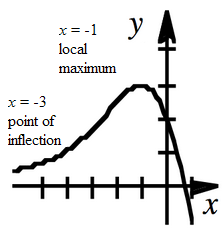### Home > APCALC > Chapter 3 > Lesson 3.3.3 > Problem3-117

3-117.

Sketch a function $f$ for which the following statements are true about its slope function $f^\prime$.

• For $x < −3, f^\prime (x) > 0$ and the slope is increasing.

• For $−3 < x < −1, f ^\prime (x) > 0$ and the slope is decreasing.

• At $x = −1, f^\prime (x) = 0$.

• For $x > −1, f^\prime (x) < 0$ and the derivative is decreasing.

Be careful! The clues give information about $f^\prime (x)$, but you are asked to sketch $f(x)$.

Translate: When $x$ is greater than $−3, f(x)$ is increasing and concave up.

Translate: When $x$ is in between $−3$ and $−1, f(x)$ is increasing and concave down.

Translate: When $x$ is exactly $−1, f(x)$ has reached a local maximum or minimum.Translate: When $x$ is greater than $−1, f(x)$ is decreasing and concave down.

Remember that this graph is based on a slope statement. Without information about the exact $y$-values of $f(x)$,the entire sketch can be shifted vertically up or vertically down.